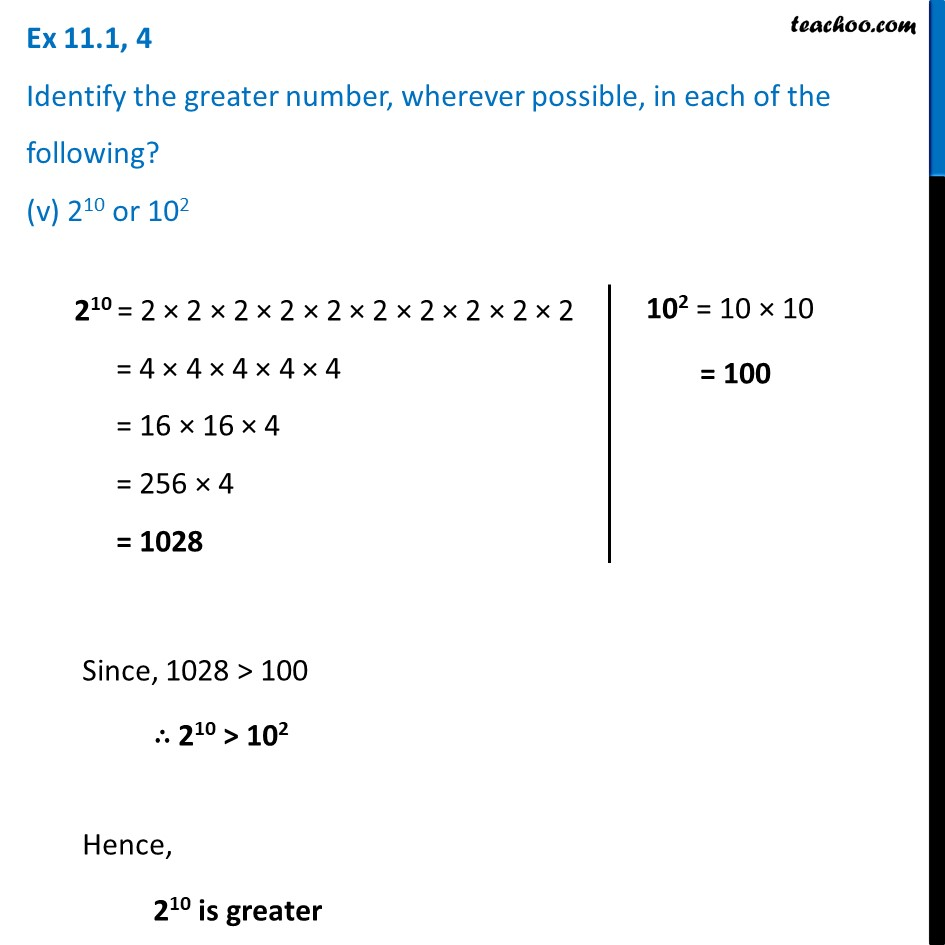Ex 11.1

Chapter 11 Class 7 Exponents and Powers
Serial order wiseLearn in your speed, with individual attention - Teachoo Maths 1-on-1 Class

### Transcript

Ex 11.1, 4 Identify the greater number, wherever possible, in each of the following? (v) 210 or 102 210 = 2 × 2 × 2 × 2 × 2 × 2 × 2 × 2 × 2 × 2 = 4 × 4 × 4 × 4 × 4 = 16 × 16 × 4 = 256 × 4 = 1028 102 = 10 × 10 = 100 Since, 1028 > 100 ∴ 210 > 102 Hence, 210 is greater